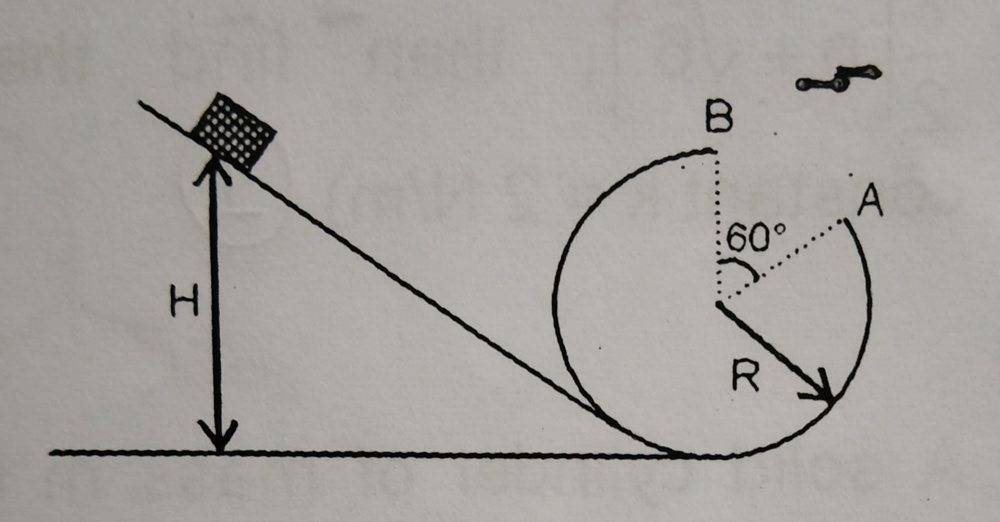# Motion in a vertical loop

Fitz Watson
Homework Statement:
A small particle slides from height H=45 cm as shown and
then loops inside the vertical loop of radius R from where a
section of angle = 60° has been removed. If R = (1/N)
meter, such that after losing contact at A and flying through
the air, the particle will reach at the topmost point B. Find
N. Neglect friction everywhere.
Relevant Equations:
$$mg(h) = mg(h') + \frac{1}{2}mv^2$$

$$mg = \frac{mv^2}{r}$$$$mg(0.45) = mg(R + R \cdot cos(\frac{π}{3})) + \frac{1}{2}mv^2$$

$$v^2 = g(0.9 - 3R)$$

The centripetal acceleration during the "flying through air" will be given by gravity

$$mg \cdot cos(\frac{\pi}{3}) = \frac{mv^2}{r}$$

$$R = \frac{1.8}{5}$$

But my book says $$R = \frac{1}{5}$$

Homework Helper
Gold Member

$$mg=\frac{mv^2}{r}$$​

is incorrect. The weight and the centripetal force do not point in the same direction. You have to consider components if this equation is meant to express Newton's 2nd law. Also, there is a normal force acting on the mass just before the particle reaches the gap.

Fitz Watson
Your equation $$mg=\frac{mv^2}{r}$$is incorrect. The weight and the centripetal force do not point in the same direction. You have to consider components if this equation is meant to be Newton's 2nd law. Also, there is a normal force acting on the mass just before the particle reaches the gap.
I've used $$mg \cdot cos\frac{\pi}{3}$$ in the solution. As for the normal force, it's not present because I've considered the instant at which the block has just left the loop

Homework Helper
Gold Member
I've used $$mg \cdot cos\frac{\pi}{3}$$ in the solution. As for the normal force, it's not present because I've considered the instant at which the block has just left the loop
At the instant at which the block has just left the loop the block is in free fall and undergoes projectile motion.

On edit: The answer in the book that you quoted is correct if the units are meters. Treat the problem as projectile motion as soon as the particle loses contact with the track.

Last edited: